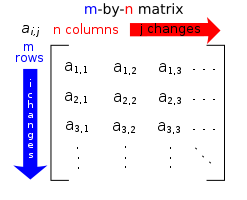indexing elements in matrix
#has-images #linear-algebra #mathematics #matricesEach element of a matrix is often denoted by a variable with two subscripts. For instance, a2,1 represents the element at the second row and first column of a matrix A.
If you want to change selection, open document below and click on "Move attachment"

Matrix (mathematics) - Wikipedia, the free encyclopedia
Matrix (mathematics) From Wikipedia, the free encyclopedia Jump to: navigation, search "Matrix theory" redirects here. For the physics topic, see Matrix string theory. <span>Each element of a matrix is often denoted by a variable with two subscripts. For instance, a2,1 represents the element at the second row and first column of a matrix A. In mathematics, a matrix (plural matrices) is a rectangular array of numbers, symbols, or expressions, arranged in rows and columns. The individual items in a matrix are called